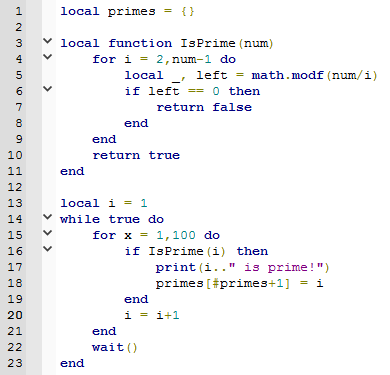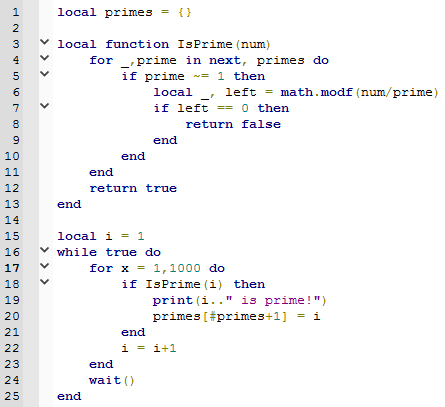# Program that calculates prime numbers.

• To calculate prime numbers, all you really have to do is check if anything besides one and itself divides evenly.

So this is a script doing that:However, you can get better than this and only check if prime numbers divide evenly into the number.This calculates 1000 numbers to determine if they're prime every 1/30 seconds. This system will begin to get laggy, though, as there are more numbers to look through each prime number you find.

• You also only need to look at the primes less than or equal to the square root of the number, and, you only need to check every odd number, adding 2 to the list of primes by default.

``````local primes = {2}
local function isPrime(num)
for i = 1, #primes do
local p = primes[i]
if num%p == 0 then
return false
elseif p*p > num then
return true
end
end
return true
end

local clock = tick()
for i = 3, math.huge, 2 do
if isPrime(i) then
primes[#primes+1] = i
if tick()-clock > 1 then
print(#primes, i)
wait()
clock = tick()
end
end
end
``````

This will calculate as many primes as it can and print how many it's found and the last one in the list every second. Finding the first million primes takes about 31 seconds.

• @1waffle1 Thanks!

The main reason why I posted this is because I knew people would help me correct my bad program.

This is very interesting.

You're very bright.

• @1waffle1 The method of only looking at odd numbers can be extended arbitrarily, too. (I think it ends up looking like the Sieve of Eratosthenes)

Consider numbers modulo 6:
if 0 mod 6, no good -- divisible by 6
if 2 mod 6, no good -- divisible by 2
if 3 mod 6, no good -- divisible by 3
if 4 mod 6, no good -- divisible by 2

Thus, we can do this

``````local primes = {2, 3, 5}
for i = 6, math.huge, 6 do
check(i + 1)
check(i + 5)
``````

This is even faster: we check 33% of numbers instead of 50% of numbers!

You can go higher by multiply the first few primes together; Let `N = 2 * 3 * 5 = 30`.

• 0 mod 30 no good
• 2 mod 30 no good
• 3 mod 30 no good
• 4 mod 30 no good
• 5 mod 30 no good
• 6 mod 30 no good
• 8 mod 30 no good
• 9 mod 30 no good
• 10 mod 30 no good
• 12 mod 30 no good
• 14 mod 30 no good
• 15 mod 30 no good
• 16 mod 30 no good
• 18 mod 30 no good
• 20 mod 30 no good
• 21 mod 30 no good
• 22 mod 30 no good
• 24 mod 30 no good
• 25 mod 30 no good
• 26 mod 30 no good
• 27 mod 30 no good
• 28 mod 30 no good

(Notice the only ones that are possibly prime are of the form `30 + p` where `p` is 1, or a prime between 5 and 30)

The above statement is sloppy as 1waffle1 pointed out. Really, `p` must be relatively prime to `30`, which is a much weaker property than primality. Since the smallest non-prime that's relatively prime to `30` is `7 * 7`, all of the numbers in the above list are prime, but in general that's not the case.

Thus we could start

``````primes = {2, 3, 5, 7, 11, 13, 17, 19, 23, 29}
for i = 30, math.huge, 30 do
check(i + 1)
check(i + 7)
check(i + 11)
check(i + 13)
check(i + 17)
check(i + 19)
check(i + 23)
check(i + 29)
``````

Now we're only checking 26% of numbers -- even better!

However, the product `N = p_1 * p_2 * p_3 * ... * p_k` grows very quickly.

For the first ten primes, `N` would be `6,469,693,230`. Thus for every six billion numbers, only several million will have to be checked. However, this only tells you how to find primes larger than 6 billion.

• Why u so smart?

Looks like your connection to Scripting Helpers was lost, please wait while we try to reconnect.